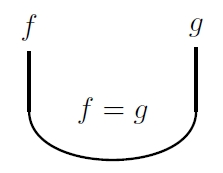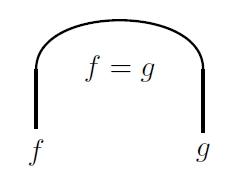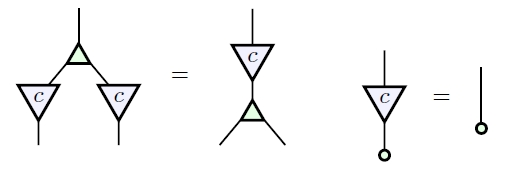# The Azimuth Project Blog - categories in control (part 2)

## Categories in Control (Part 2): LinRel and Corelations

Today we’ll philosophise about representing systems with category theory.

In the previous post in this series, John and Jason gave a presentation of the category of $FinRel_k$ of linear relations. Let’s remind ourselves of the generators:Here $c$ is an element of the field $k$.

The equations take quite a bit longer to write out: there are 31 of them! This seems a large number, but they’re pretty easy to remember once you get the hang of it. We build them from simpler, more well-known structures. For example, the black multiplication and black unit form a commutative monoid:And the white comultiplication and white counit form the mirror image – a cocommutative comonoid:Together they form a bimonoid:And the scalars are homomorphisms for our monoid and comonoid:These 14 equations, together with the generators that appear in them, axiomatise the category $FinVect_k$ of vector spaces and linear maps. But we’re still short 17 equations, and 2 generators, of our presentation the category of vector spaces and linear relations. How do we understand the additional ones?

Like any superhero, and linear algebra certainly does more than its fair share of work, the best way to understand the motivation here is a good origin story. And like all the best superheroes, linear algebra has a bunch of good origin stories. Let’s take a look at a new one, about how linear relations come to be. It involves many of our favourite ideas, like cospans and corelations.

(Remember, corelations are dual to binary relations, and have little relationship to correlations.)

### Combining systems

Our story begins with that great sage of category theory: the Yoneda lemma. Yoneda’s lemma has many faces, but one of its deepest insights is that an object is no more, nor less, than its web of relationships.

Suppose we interact with a linear system $V$ by taking some measurement device $T$, also a linear system, and representing $V$ in $T$. This means we interact with $V$ via linear maps $V \to T$, and $\hom(V,T)$ provides a complete description of how we can understand $V$ with $T$.

Now, if we have two systems $V$ and $W$, this means we understand them together via pairs of maps, one from $\hom(V,T)$, and one from $\hom(W,T)$. If we wanted to think of them together as a single linear system $U$, then $\hom(U,T)$ would have to naturally be in bijection with the set $\hom(V,T) \times \hom(W,T)$. But this is the definition of coproduct! So the combined system $U$ is precisely the coproduct of vector spaces $V \oplus W$. More generally, to combine interacting systems, we take the colimit of the diagram depicting the systems and their interactions.

A great setting for reasoning about colimits is the category of cospans! Remember that a cospan is a pair of maps $A \to S \leftarrow B$: the category of cospans has the same objects as the original category, but has cospans instead of the usual maps as morphisms. The monoidal structure is the coproduct, and composition is by pushout.

Now a theorem of Mac Lane says that if a category has both coproducts and pushouts, then it has all finite colimits: you can use coproducts and pushouts to construct them all. So you should be able to construct these finite colimits in the monoidal category of cospans. Rosebrugh, Sabadini, and Walters work out some of the details here:

• Robert Rosebrugh, Nicoletta Sabadini, and Robert F.C. Walters, Calculating colimits compositionally, in Concurrency, Graphs and Models, Lecture Notes in Computer Science 5065, pp. 581-592, Springer, 2008.

From this perspective, a linear system might be represented by a cospan $A \to S \leftarrow B$ in the category of linear maps. The feet $A$, $B$ of the cospan represent the boundary of the system, and the maps to the apex $S$ represent how the boundary includes into the larger system. Composition in the category of cospans models connecting one system with another along a common boundary.

### Understanding boundaries

Let’s spend some time exploring what the boundary means. First, the boundary provides a way to connect one system with another. Given systems $A \to S \leftarrow B$ and $B \to R \leftarrow C$, we can compose these cospans to get a system with boundary $A$ and $C$.

Second, the boundary models our access to the system; we think of the apex system as being enclosed in a black box, with the boundary our only channels in and out of the system. This implies we shall only consider systems different if we can see that difference from the boundary.

Write $B$ for the boundary of a system $S$, so that we have a map $B \to S$ in our category. Again, to study $S$ we are interested in the maps from $S$ to $T$. But remember that $S$ is internal to the black box; we can only witness the induced maps from the boundary $B \to S \to T$.

In the category of vector spaces and linear maps, such maps $B \to T$ induced by maps $S \to T$ are in one-to-one correspondence with maps $S' \to T$, where $S'$ is the unique up-to-isomorphism object such that $B \to S$ factors $B \to S' \to S$, where the first map is an epimorphism and the second map a monomorphism. This means that, without loss of generality, we may assume our systems to be epimorphisms $B \to S$.

In the world of cospans, this means we consider only jointly-epic cospans. These are known as corelations!

### Corelations in $FinSet$

As a warm-up example, let’s revisit the category of corelations in $FinSet$.

We’ll think of a finite set as a collection of copper wires. A cospan tells us how we can connect these wires with other wires. So we can visualise the cospan

as modelling the wire network

.

But if this network of wires is in a black box, we’ll never be able to interact with the parts that are completely enclosed. So this network is equivalent to the network

which we model with the corelation:

This is a corelation because every point in the apex is mapped to by a point in the boundary. This jointly-epic condition means that we only care about networks that touch the boundary: if you can’t connect anything to a network that lives inside the box, then it may as well not be there!

If you want to explore this idea further, check out

### Linear relations as corelations in $FinVect_k$

So, back to our initial question. How do understand the equations that present $FinRel_k$? If the morphisms of $FinRel_k$ model linear systems with boundary, then our philosophy suggests they should be the equations that characterise corelations in $FinVect_k$. Our philosophy checks out: the category of corelations in $FinVect_k$ is equivalent to $FinRel_k$!

An alternate axiomatization involves more generators, based on this idea. Take the generators of $FinVect_k$ and their mirror images. We don’t include the cup and cap as generators, because they then can be constructed as follows:

The axioms include all the mirror images of the relations for $FinVect_k$, since we’re now allowed to write our maps backwards. So the black mirror image generators now form a cocommutative comonid

and so on. To derive the rest of the equations, we first compute pushouts of all the generators in $FinVect_k$. Bonchi, Sobocinski, and Zanasi do this in

They then show you get almost all the equations you need from these pushouts: you’ll be missing just one! The missing one is the (dark) extra law:

By using an argument similar to the one that was used with Brandon in our paper linked above, you can check that the extra law is the only additional law you need to axiomatize corelations from cospans!

It’s not hard to show that this also is a presentation of $FinRel_k$: we just check that all of John and Jason’s equations can be derived from the BSZ equations, and vice versa.